Question

# Glucose solutions used for intravenous medical applications are typically 5.00 % (m/m) Glucose dissolved in water....

Glucose solutions used for intravenous medical applications are typically 5.00 % (m/m) Glucose dissolved in water. What is the molarity associated with a typical 5.00 % Glucose solution. Molar Mass (Glucose) = 180.16 g/mol; Density of normal glucose solution = 1.01 g/mL

1. 0.280 M             b) 0.364 M                 c) 2.75 M                   d) 3.57 M                   e) 9.09 M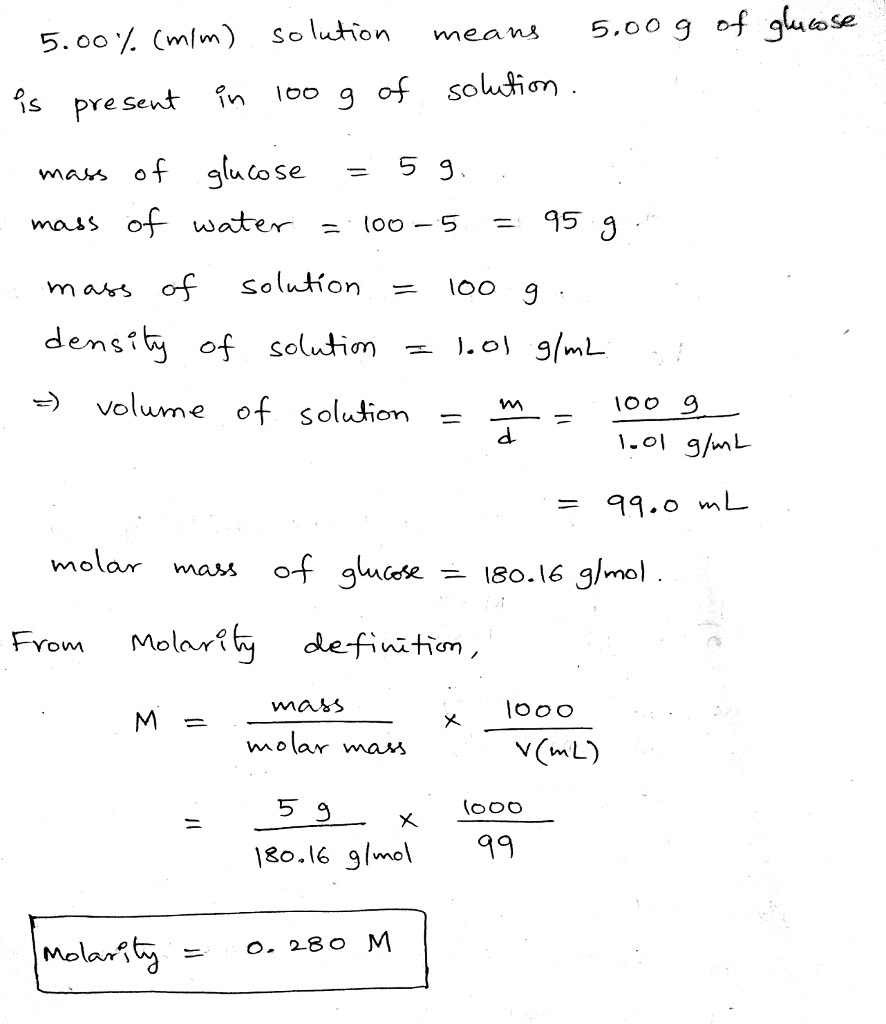#### Earn Coins

Coins can be redeemed for fabulous gifts.

Similar Homework Help Questions
• ### 1. Glucose (C6H12O6, M= 180.16 g/mol) solutions are commercially available. A concentrated sample of an aqueous...

1. Glucose (C6H12O6, M= 180.16 g/mol) solutions are commercially available. A concentrated sample of an aqueous glucose solution has a density of 1.25 g/mL and contains 55.0 % glucose by mass at 20 °C a) What is the molarity of this glucose solution? b) What is the molality of this solution? c) What is the mole fraction of glucose in this solution?

• ### 6) Two solutions are prepared. The first contains glucose (C.H.O.) dissolved in water at 0.180 M...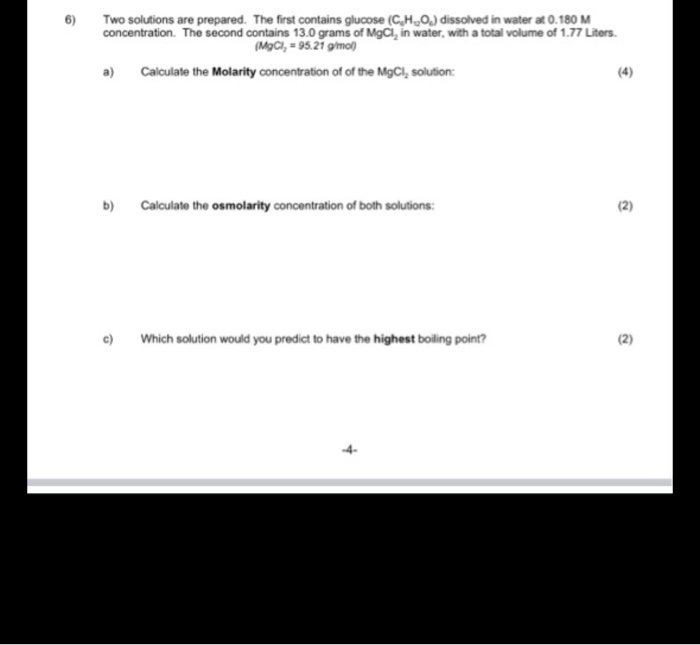6) Two solutions are prepared. The first contains glucose (C.H.O.) dissolved in water at 0.180 M concentration. The second contains 13.0 grams of MgCl, in water, with a total volume of 1.77 Liters. (MgCl, = 95.21 g/mol) a) Calculate the Molarity concentration of of the MgCl, solution: b) Calculate the osmolarity concentration of both solutions: 12) Which solution would you predict to have the highest boiling point? (2)

• ### 8. Which beaker contains more dissolved glucose particles? Solution A Solution B 50.0 mL of 1.25...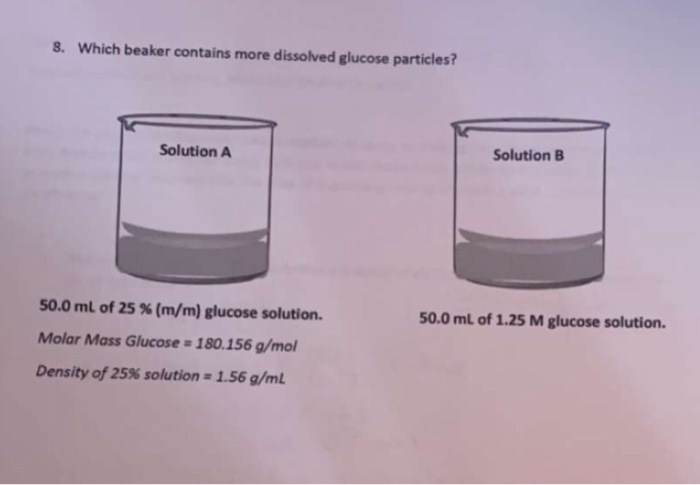8. Which beaker contains more dissolved glucose particles? Solution A Solution B 50.0 mL of 1.25 M glucose solution. 50.0 mL of 25 % (m/m) glucose solution. Molar Mass Glucose - 180.156 g/mol Density of 25% solution = 1.56 g/mL

• ### 6) Two solutions are prepared. The first contains glucose (C,H,O) dissolved in water at 0.180 M...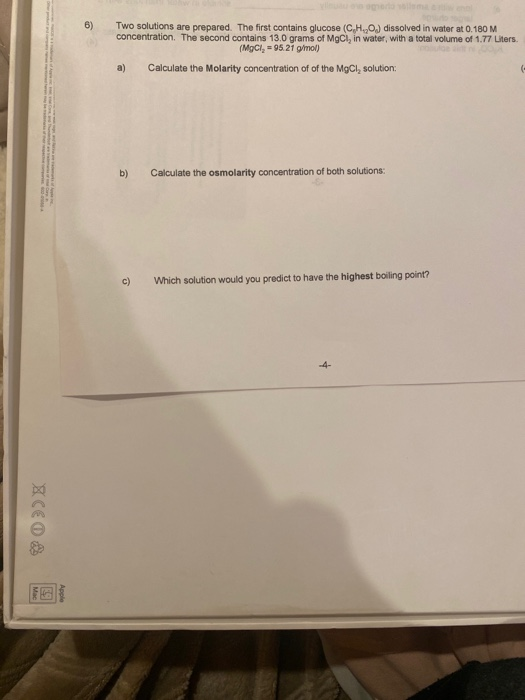6) Two solutions are prepared. The first contains glucose (C,H,O) dissolved in water at 0.180 M concentration. The second contains 13.0 grams of MgCl, in water, with a total volume of 1.77 Liters. (MgCl, = 95.21 g/mol) a) Calculate the Molarity concentration of of the MgCl, solution: b) Calculate the osmolarity concentration of both solutions: c) Which solution would you predict to have the highest boiling point? 수 XCEO

• ### 3. A glucose solution (CHO) is prepared by adding 5.00 g of glucose to enough water...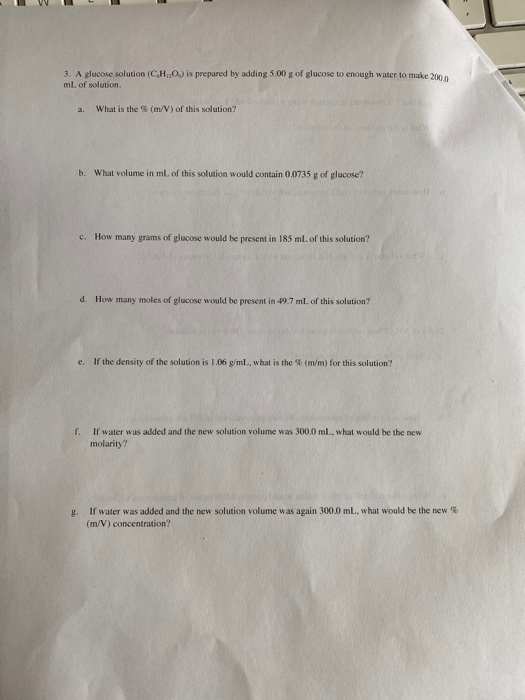3. A glucose solution (CHO) is prepared by adding 5.00 g of glucose to enough water to make 2000 mL of solution What is the % (m/V) of this solution? b. What volume in ml. of this solution would contain 0.0735 g of glucose? c. How many grams of glucose would be present in 185 ml of this solution? d. How many moles of glucose would be present in 49.7mL of this solution? e. If the density of the solution...

• ### 6. 2.50 grams of nicotine (C10H14N2) was dissolved in 150.0 g of dichloromethane. What is the...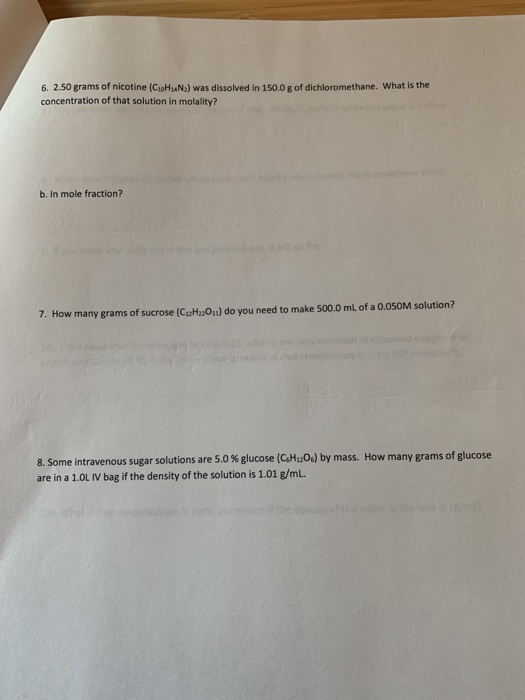6. 2.50 grams of nicotine (C10H14N2) was dissolved in 150.0 g of dichloromethane. What is the concentration of that solution in molality? b. In mole fraction? 7. How many grams of sucrose (C12H220.1) do you need to make 500.0 mL of a 0.050M solution? 8. Some intravenous sugаr solutions are 5.0% glucose (C.H1206) by mass. How many grams of glucose are in a 1.0L IV bag if the density of the solution is 1.01 g/mL.

• ### A 5.00 g quantity of a diprotic acid (H2A) was dissolved in water and made up...

A 5.00 g quantity of a diprotic acid (H2A) was dissolved in water and made up to exactly 250 mL. Calculate the molar mass (in g/mol) of the acid if 25.0 mL of this solution reuired 11.1 mL of 1.00 M KOH for neutralization.

• ### Determining the Concentration of a BaC12 Solution A 5.00 mL solution of BaCl2 dissolved in water...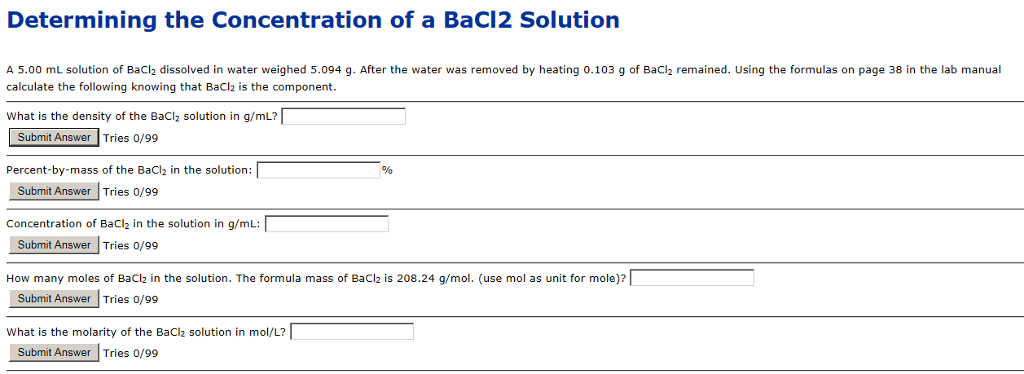Determining the Concentration of a BaC12 Solution A 5.00 mL solution of BaCl2 dissolved in water weighed 5.094 g. After the water was removed by heating 0.103 g of BaCl2 remained. Using the formulas on page 38 in the lab manual calculate the following knowing that BaCl2 is the component. What is the density of the BaCl2 solution in g/mL? ubmit Answer Tries 0/99 Percent-by-mass of the BaCl2 in the solution: Stlemit Answer lTrie /9 Concentration of BaCl in the...

• ### The total concentration of dissolved particles in blood is 0.30 M. An intravenous (IV) solution must...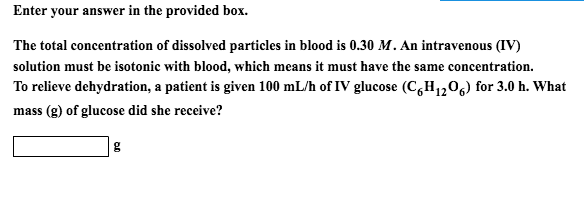The total concentration of dissolved particles in blood is 0.30 M. An intravenous (IV) solution must be isotonic with blood, which means it must have the same concentration. To relieve dehydration, a patient is given 100 mL/h of IV glucose (C_6H_12O_6) for 3.0 h. What mass (g) of glucose did she receive? g

• ### commercial grade HCL solutions are typically 39.0% (by mass) HCL in water. Determine the molality and molarity of the H...

commercial grade HCL solutions are typically 39.0% (by mass) HCL in water. Determine the molality and molarity of the HCL, if the solution has a density of 1.20 g/ml.